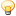CodeGuru Home VC++ / MFC / C++ .NET / C# Visual Basic VB Forums Developer.com

# Thread: Newtons Method for Approximating Roots

1. ##Newtons Method for Approximating Roots

Hi, i have this method here that approximates square roots. I was wondering what ppl thought about it and whether it is following a correct algorithm. It seems to work good and gave pretty close approximations for all my test casses. The only issue is on the very first call to the function, if 1 is passed in as a guess it returns bogus numbers.

Code:
```/*
* Newtons Method for Approx. square roots.
* Accepts 2 doubles.
* Argument 1 is the number to take the square root of.
* Argument 2 is the approximation/guess of the square root.
* x =  1/2( x + A/x)  <-- Formula being used.
*/
double approxSQRT(double A, double approx)
{
double previous=approx;

approx = (0.5)*(approx + ( A/approx));

//When sequential approximations have a negligable difference the
//   approximation should be very close to the actual square root.
if(previous - approx < 0.0000001 )
return(approx);

return( approxSQRT(A,approx));
}```Reply With Quote

2. ## Re: Newtons Method for Approximating Roots

The full driver prog.

Code:
```/*
Gerald Eckert

Using Newtons Method for Approximating Roots

Formula.
x =  1/2( x + A/x)

x represents the square root or the approximation.

Continue running through this formula untill the
difference in sequential x values becomes negligable.
*/

#include <iostream>
using namespace std;

double approxSQRT(double,double);

int main()
{
double numA=0;     // numA represents the number under the square root.
double guess=0;    // guess represents the users guess at the square root.
double sqrt=0;

cout<<"Take the square root of what number? : ";
cin>>numA;

cout<<"Please take a guess at the square root of "<<numA<<" : ";
cin>>guess;

sqrt= approxSQRT(numA,guess);

cout<<"The square root of "<<numA<<" is approximatly "<< sqrt <<endl;

return(0);
}

/*
* Newtons Method for Approx. square roots.
* Accepts 2 doubles.
* Argument 1 is the number to take teh square root of.
* Argument 2 is the approximation/guess of the square root.
* x =  1/2( x + A/x)  <-- Formula being used.
*/
double approxSQRT(double A, double approx)
{
double previous=approx;

approx = (0.5)*(approx + ( A/approx));

//When sequential approximations have a negligable difference the
//   approximation should be very close to the actual square root.
if(previous - approx < 0.0000001 )
return(approx);

return( approxSQRT(A,approx));
}```Reply With Quote

3. ## Re: Newtons Method for Approximating Roots

I was doing some more testing and found some weird bugs. The bugs pop up when a user would enter really bad guesses. Like if they guess 34030 for the square root of 125. If anyone knows the algorithm would you let me know if i am using it right. ThanksReply With Quote

4. ## Re: Newtons Method for Approximating Roots

It returns bogus answers. Like I try approxSQRT(555 , 3) and it returns 94. Ill have to do the math by hand and see what is going on.Reply With Quote

5. ZuKSenior MemberJoin Date
Oct 2002
Location
Austria
Posts
1,284

## Re: Newtons Method for Approximating Roots

Your program only works if the initial guess is above the result.

try
Code:
`     if(fabs(previous - approx) < 0.0000001 )`
KurtReply With Quote

6. ## Re: Newtons Method for Approximating Roots

Ok, stupid error on my part.
Code:
```
approxSQRT(555 , 3)

use the formula

(1/2)*(3 + 555/3)  =  94

find the difference

3 - 94 =  - 91

compare the difference

is  -91 < 0.000001    //Should be using absolute value here.

XD..```
I think using absolute value would solve the issue.

Thanks ZuK, was writing this when you repliedReply With Quote

7.Member +Join Date
Jan 2009
Posts
596

## Re: Newtons Method for Approximating Roots

It would make more sense to use an iterative method here, rather than recursion. With recursion it would be possible to get a stack overflow.

Code:
```double approxSQRT(double A, double approx)
{
double previous = 0.0;
do {
previous = approx;
approx = (0.5)*(approx + (A/approx));

} while( fabs(previous - approx) < 0.0000001 );

return approx;
}```
Or you might want to change the while condition to keep going until the actual value is close enough to the square root, rather than just not changing by much, i.e.

Code:
`while( fabs( (approx * approx) - A ) < some constant )`Reply With Quote

algorithm, math, newton, recursive, square roots####Posting Permissions

• You may not post new threads
• You may not post replies
• You may not post attachments
• You may not edit your posts
•